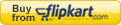# [Studyplan] ACIO: Aptitude, Maths, Reasoning and English Essay for Assistant Central Intelligence Officer (ACIO) exam

## [Studyplan] ACIO: Aptitude, Maths, Reasoning and English Essay for Assistant Central Intelligence Officer (ACIO) exam

1. Prologue
2. Maths / Quantitative Aptitude
1. Probability
2. Algebra, Linear Eq.
3. Average- Allegation
4. Time speed distance work
5. Percentage, profit-loss, SI-CI
6. Number theory, LCM-HCF
7. AVP (Area Volume Perimeter)
3. Reasoning
1. #1: Chart based
2. #2: coding n series + alphabet
3. #3 Analogy, odd man
4. Non-priority
4. English

# Prologue

In the Assistant Central Intelligence Officer (ACIO) exam, you’ve to face two papers

1. Paper I: 100 Multi-choice questions from General Awareness + aptitude (=Maths + reasoning)
2. Paper II: English Essay worth 50 marks

Total time limit: 1 hr 40 minutes

In this article, we see the approach for Maths, reasoning and English.

The approach for General awareness (History, geography, science, current affairs etc.) is given in a separate article: click me

In ACIO 2012 Question paper, the internal breakup was:

 General Awareness 79 Maths 9 Reasoning 12 Total MCQ 100

Therefore, Maths+ Reasoning=9+12=21= approx. 1/5th of paper was about Aptitude. So cost:benefit is quite good but Practice@home=essential, otherwise following things will happen:

## #1: silly mistakes in calculation

 wrong right x-5=(15/2)2*x-5=152x=15+52x=20 x=10 x-5=15/22(x-5)=152*x-(2*5)=152x-10=15 2x=25 x=12.5

## #2: formula problem

you won’t be able to recall formulas or you will plug wrong values in the formula=> either way answer will not come. (Example: area of equilateral triangle asked in ACIO-2012)

## #3: time problem

• In maths, Because of problem #1 + #2= your answer will not match the given options A/B/C/D then you re-calculate the whole sum again=time wasted.
• In reasoning, you’ll take lot of time in each sitting-arrangement/blood-relation / coding-decoding type question. = time wasted

In short, if you want to crack a government / Bank / PSU exam=> aptitude practice@home is essential.

# Maths / Quantitative Aptitude

Donot Fear Maths, Donot Hate Maths. If you basic concepts are clear and you practice enough at home, then most of maths/reasoning questions can be solved without effort or mistake during the exam.

Negative thinking “maths is too difficult, ye mujse nahi hoga”=> you’ll only dig your own grave while others prepare maths and get selected for the job.

For all competitive exams of India, we can classify math topics into following

 Regular topics SSC/CDS/CAT exclusive Misc Topics* prepare for ACIO Ignore for ACIO prepare for ACIO basics (bodmas, surds-indices etc) Number theory, HCF, LCM Algebra, linear equation ratio-proportion-variation average, allegations %, profit loss, SI-CI (interest rate) Time-speed Distance and work (TSDW):includes pipe-cistern, boats streams AVP (Area-volume perimeter) Geometry other than AVP coordinate geometry trigonometry quadratic equations logarithms (Usually non-SSC/CDS/CAT exam don’t bother much asking questions from this topics). PCP (Permutation-Combination-Probability) AP-GP (Arithmetic progression, geometric progression) (Most govt-job exams nowadays don’t bother with these topics but they were asked in ACIO-2012 hence need to be prepared.)

## Preparation Source:

2. Mrunal.org/aptitude
3. Any one Quantitate Aptitude book. Even second hand /used copy will work- you don’t need latest edition. Based on their utility for SSC-CGL exam, I classify the Quant books into 3 types:
 will work in ACIO + Useful for SSC-CGL; CDSBecause quadratic eq., trigonometry and geometry-theory (non-AVP) is covered properly.Fast-track to Object Arithmetic (Rajesh Verma) Sarvesh Kumar (Quantam CAT) will work in ACIO but Not so useful for SSC-CGL; CDSBecause quadratic eq., trigonometry and non-AVP geometry is not covered in length. M.Tyra Quicker Maths (BSC publication) R.S.Agarwal Quantitative Aptitude Useless for any exam Readymade Junk from jholachhap publishers like “ACIO guide book; RBI assistant guidebook; National Insurance AO guidebook” etc.
1. For additional practice you may go through previous SSC question papers (but ignore trigonometry, quadratic equation and geometry except Area-volume-perimeter.)

## Permutation combination probability (PCP)

In the late-90s, this used to be the hot-favorite topic for govt.exams, but nowadays rarely comes. However ACIO-2012 did contain two questions from PCP (meaning ACIO paper-setter uncleji still runs with 90s mindset) so you’ve to prepare PCP. Most of the PCP stuff can be solved without any formulas. Go through following articles

1. [Aptitude] Permutation Combination made easy without Formulas
2. [Aptitude] Combination: Coins possible Sums (without formula)
3. [Aptitude] PnC: arrange such that two people Always sitting together?
4. [Aptitude] PnC: How many Ways to arrange letters of word “RECUPERATE” (Permutation without Formulas)
5. [Aptitude] PnC Arrange digits in ascending order to form numbers (asked in UPSC 2009)
6. [Aptitude] PnC: Conditional Selection in Committee
7. [Aptitude] PnC: Binomial Expansion. How many ways to select one or more out of given Items?